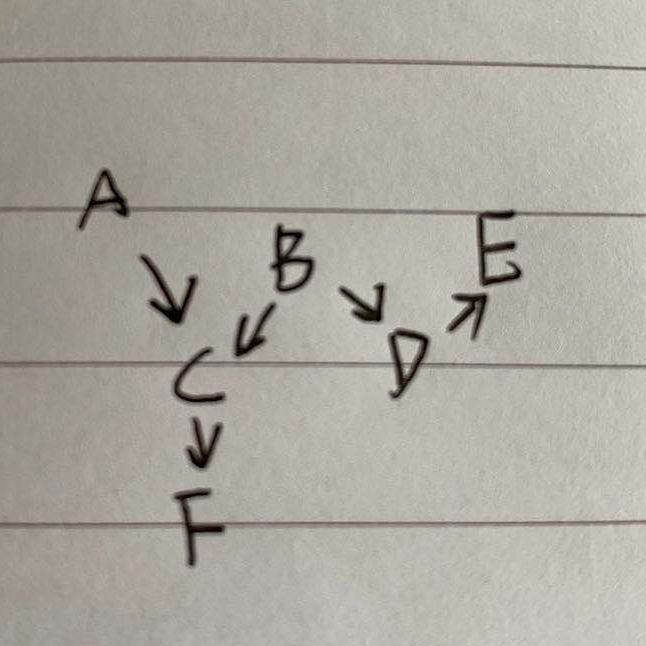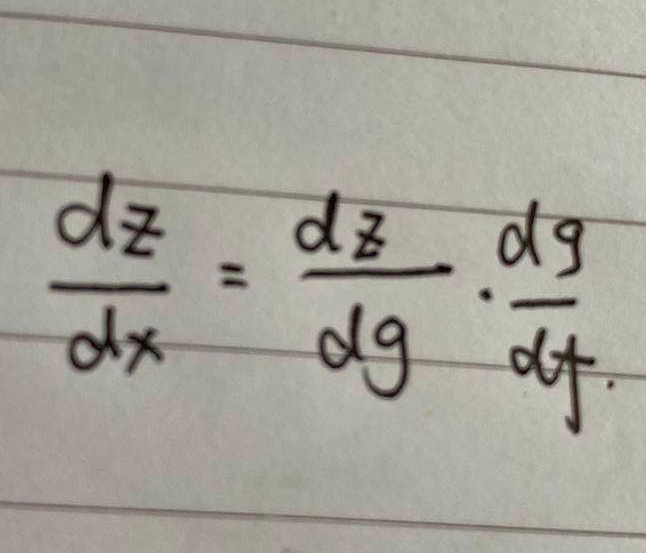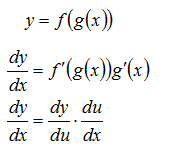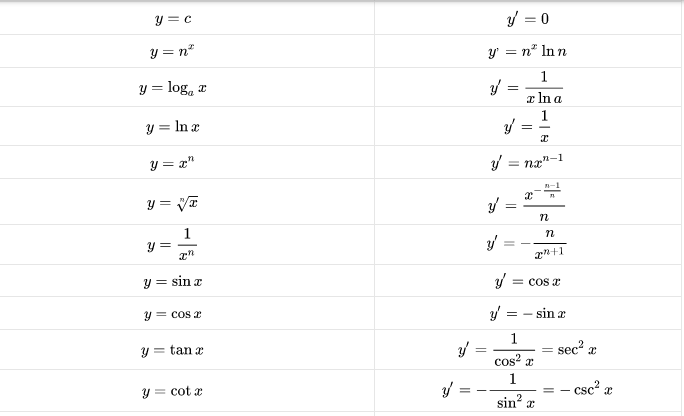• AI基础：链式法则、幂法则、广义幂法则
2021-09-07 18:29:42

概念回顾

# 幂法则

有时候也会叫次幂法则，仍然是函数求导的法则

就是幂次函数的求导法则
f ( x ) = x 2 的 导 数 f ( x ) ‘ = ( x 2 ) ‘ = 2 x f\left(x\right)=x^2的导数f\left(x\right)^=\left(x^2\right)^=2x

# 链式法则(Chain Rule)

求复合函数导数的一个法则, 是微积分中最重要的法则之一

两个函数组合起来的复合函数，导数等于里面函数代入外函数值的导乘以里面函数之导数

公式表示如下：
y = f ( g ( x ) ) d y d x = f ‘ ( g ( x ) ) g ‘ ( x ) 另 一 种 形 式 ： d y d x = d y d u . d u d x y=f\left( g\left( x \right) \right) \\ \frac{dy}{dx}=f^\left( g\left( x \right) \right) g^\left( x \right) \\ 另一种形式： \frac{dy}{dx}=\frac{dy}{du}.\frac{du}{dx}

其实就是把g(x)看作一个整体

## 示例

求 f ( x ) = sin ⁡ ( x 2 + 1 ) 的导数 这个函数可以看成 f ( x ) = sin ⁡ ( g ( x ) ) , 其中 g ( x ) = x 2 + 1 求解就套入公式： f ( x ) ‘ = sin ⁡ ( g ( x ) ) ‘ ∗ g ( x ) ‘ = cos ⁡ ( g ( x ) ) ∗ 2 x = 2 x cos ⁡ ( x 2 + 1 ) \text{求}f\left( x \right) = \sin \left( x^2 + 1\right) \text{的导数} \\ \text{这个函数可以看成}f\left( x\right) = \sin \left( g\left(x \right)\right),\text{其中}g\left( x\right)=x^2+1 \\ \text{求解就套入公式：}f\left(x\right)^=\sin \left(g \left(x\right) \right)^ * g\left(x\right)^ \\ =\cos \left(g\left(x\right)\right)*2x \\ =2x\cos\left(x^2+1\right)

另一种形式的话，上述例子就是所谓的u，一样的理解

## 链式法则的应用

• 在机器学习领域，链式法则是需要理解的重要基础法则之一，其主要应用在于反向传播（backpropagation）等算法

反向传播算法是在模式识别和故障诊断等领域广泛使用的简单方法

• 目前关于神经学习的研究有很多，但其主要研究方向在于在新领域应用神经网络，或具有更好表现的神经网络，其训练算法依赖链式法则这一本质是没有变过的。

• 链式法则在反向传播算法中，存在学习效率低下，收敛速度慢，缺乏仿生学依据等问题

• 目前机器学习社区正在大力发展无监督学习，而无监督学习并不依赖于链式法则这类有监督的算法

# 广义幂法则

其实就是链式法则去理解，应用幂法则求导。就是链式法则+幂法则

含义和链式法则差不太多，都是把部分看作整体
公式： d y d x u n = n u n − 1 d u d x 示例：求 y = ( x 2 + 1 ) 3 的导数 根据广义幂法则公式： d y d x = 3 ( x 2 + 1 ) 2 . d ( x 2 + 1 ) d x = 3 ( x 2 + 1 ) 2 . x 2 \text{公式：}\frac{dy}{dx}u^n=nu^{n-1}\frac{du}{dx} \\ \text{示例：求}y=\left( x^2+1\right)^3\text{的导数} \\ \text{根据广义幂法则公式：}\frac{dy}{dx}=3\left( x^2+1\right)^2.\frac{d\left( x^2+1\right)}{dx}=3\left( x^2 + 1\right)^2.x2神经网络 深度学习 机器学习
更多相关内容
• ## 链式法则

千次阅读 2020-12-22 20:41:56
在微积分中，链式法则是计算两个或多个函数的组合的导数的公式，也就是说，如果f和g是函数，则链式法则表示它们的组合的导数f∘g(将x映射到f(g(x))的函数。由于在定义过程中求导公式可以表示成一个连乘过程，就像...

在微积分中，链式法则是计算两个或多个函数的组合的导数的公式，也就是说，如果f和g是函数，则链式法则表示它们的组合的导数f∘g(将x映射到f(g(x))的函数。由于在定义过程中求导公式可以表示成一个连乘过程，就像锁链一样一环套一环，故而得名。链式法则在计算上简单，在直观上容易理解。下面我们给出正式定义。

设f和g为两个关于x的可导函数，则复合函数$(fog)'(x)$的导数为：

$$(fog)'(x)=f'(g(x))g'(x)$$如$g(x)=x^2+1$，$h(g)=g^3$，则求导$h(g(x))=g(x)^3$时计算过程应为：

$f'(x)=[h(g(x)]'=3(g(x))^2(2x)=3(x^2+1)^2(2x)=6x(x^2+1)^2$

当函数扩展为多元复合函数$z=f(x,y)$，其中$x=g(t), y=h(t)$且h(t)和g(t)可微，则：

dz/dt=\partial z/\partial x dx/dt+\partial z/\partial y dy/dt描述

在机器学习领域，链式法则是需要理解的重要基础法则之一，其主要应用在于反向传播(backpropagation)等算法，反向传播算法是在模式识别和故障诊断等领域广泛使用的简单方法。其发明者Werbos于1990年再次讨论反向传播所基于的定理——有序导数(ordered derivatives)的链式法则，并设计了可以转换为计算机代码，并由神经网络用户直接应用的更简单的反向传播版本。

目前关于神经学习的研究有很多，但其主要研究方向在于在新领域应用神经网络，或具有更好表现的神经网络，其训练算法依赖链式法则这一本质是没有变过的。

主要事件年份事件相关论文/Reference

1975Werbos发明的反向传播算法Werbos, P.J. (1975). Beyond Regression: New Tools for Prediction and Analysis in the Behavioral Sciences.

1990Werbos再次讨论反向传播所基于的定理——有序导数(ordered derivatives)的链式法则，并设计了可以转换为计算机代码，并由神经网络用户直接应用的更简单的反向传播版本Werbos, P.J. (1990). Backpropagation through time: what it does and how to do it.Proceedings of the IEEE. 78(10):1550 - 1560.

1993Jang提出的ANFIS(adaptive-network-based fuzzy inference system)体系结构和学习过程中对残差的计算仍然是基于链式法则的Jang J. S. R. (1993). ANFIS: adaptive-network-based fuzzy inference system. IEEE Transactions on Systems, Man, and Cybernetics. 23(3): 665 - 685.

发展分析

瓶颈

链式法则是一种在数学成立上的求导法则，并不像技术和算法等有适用范围和瓶颈。但在其实际应用中，仍以反向传播算法为例，往往存在学习效率低下，收敛速度慢，缺乏仿生学依据等问题。此外，目前机器学习社区正在大力发展无监督学习，而无监督学习并不依赖于链式法则这类有监督的算法。

未来发展方向

Contributor: Yuanyuan Li

展开全文• 本篇介绍了微积分链式法则和概率链式法则。 一、概率链式法则 这里首先给出概率链式法则的公式：N个事件的概率链式法则如下， P(X1, X2, ... Xn) = P(X1 | X2, X3 ... Xn) * P(X2 | X3, X4 ... Xn) ... P(Xn-1 | ...

本篇介绍了微积分链式法则和概率链式法则。

# 一、概率链式法则

这里首先给出概率链式法则的公式：N个事件的概率链式法则如下，
P(X1, X2, ... Xn) = P(X1 | X2, X3 ... Xn) * P(X2 | X3, X4 ... Xn) ... P(Xn-1 | Xn) * P(Xn)。

概率链式法则的作用特别体现在，当我们的事件存在独立相关性的情况下。比如说，当A和B两个事件相互独立时，P(A|B)=P(A)。
当A、B相互独立，并把它推广到三个事件时，有P(A|B,C)=P(A|C)=P(B|C)。其中P(A|B,C)表示在B和C事件都发生的情况下，A事件发生的概率。由条件概率（P(A|B)）的定义我们知道，条件概率是指事件A在另外一个事件B已经发生条件下的发生概率。这里既然A与B相互独立，那B就不是A是否发生的条件，A就只与C有关。假设有事件ABCDEF，箭头表示它们之间存在的依赖关系，其中F只和C有关，E只和D有关，D只和B有关，C和A、B有关。假设这里要求ABCEDF事件同时发生的概率P（A,B,C,D,E,F）是多大？
这里完整的公式是这样的：
P（A,B,C,D,E,F）=P（F|A,B,C,D,E）*P（A,B,C,D,E）
=P（F|A,B,C,D,E）*P（C|A,B,D,E）*P（A,B,D,E）
=P（F|A,B,C,D,E）*P（C|A,B,D,E）*P（E|A,B,D）*P（A,B,D）
=P（F|A,B,C,D,E）*P（C|A,B,D,E）*P（E|A,B,D）*P（D|A,B）*P（A,B）
=P（F|A,B,C,D,E）*P（C|A,B,D,E）*P（E|A,B,D）*P（D|A,B）*P（A|B）*P（B）

但是利用事件的独立性，上面复杂的式子可以简化为：
P（A,B,C,D,E,F）=P（F|C）*P（C|A,B）*P（E|D）*P（D|B）*P（A）*P（B）

事件的独立性：

1.F只和C有关，P（F|A,B,C,D,E）=P（F|C）
2.C只和A、B有关，P（C|A,B,D,E）=P（C|A,B）
3.A和B无关，P（A|B）=P（A）
4.E只和D有关，P（E|A,B,D）=P（E|D）
5.D只和B有关，P（D|A,B）=P（D|B）

# 二、微积分链式法则

微积分链式法则主要是用于计算复合函数的导数。在BP算法中，我们就会用到微积分链式法则，这是一种高效的特定运输顺序。下面是problem formulation：
假设实数x，f(·)和g(·)是从实数映射到实数的函数。若y=f(x)，z=g(f(x))，则微分链式法则为：展开全文• ## 链式法则 理解应用

千次阅读 2021-07-29 20:59:23
链式法则是微积分中的求导法则，用于求一个复合函数的导数，是在微积分的求导运算中一种常用的方法。复合函数的导数将是构成复合这有限个函数在相应点的 导数的乘积，就像锁链一样一环套一环，故称链式法则。 为啥提...

链式法则是微积分中的求导法则，用于求一个复合函数的导数，是在微积分的求导运算中一种常用的方法。复合函数的导数将是构成复合这有限个函数在相应点的 导数的乘积，就像锁链一样一环套一环，故称链式法则。

为啥提链式法则，因为这对深度学习，神经网络的参数求解计算起着绝对的影响作用，之前提过神经网络中基本使用了梯度下降法进行参数优化求解，具体关于神经网络的基本组成单元感知机，神经网络是很多感知机根据复杂的连接关系构成，所以详细可以看看感知机的梯度下降求解参数过程就能知道神经网络中每个感知机的参数求解了。

因为感知机的函数关系很简单，可以直接求解，但是神经网络是很多层感知机连接在一起，第一层感知机把数据信息传递给下一层，于是这样的关于我们最终的损失函数Loss和第一层或者第二层（针对多层神经网络）的感知机的参数求解就很麻烦了，于是我们需要利用微积分中的链式法则求解层层参数关系的导数计算。

## 一、链式法则

链式法则是将复杂函数进行简单化，其基本表达关系如下：## 二、举个栗子

比如函数

y=(2x+e^x)^2


关于y的导函数的计算，咋一看好像很复杂，但根据链式法则就是

y=u^2
u=2x+e^x
dy/du=2u
du/dx=2+e^x
于是
dy/dx=(dy/du)*(du/dx)
=(2u)*(2+e^x)
=(2*(2x+e^x))*(2+e^x)
`

这样就是把复杂函数化简成我们熟知的常见导函数形式。

## 三、常见的导函数求解公式表

顺便贴一下。展开全文神经网络 深度学习 人工智能 机器学习 python
• 链式法则 至于复合函数的求导公式为什么称为链式法则，原因是如果把表示过程用导数的另外一种符号表示如下: dy=dy du du=dy dudu dx dx\mathrm{d} y=\frac{\mathrm{d} y}{\mathrm{~d} u} \mathrm{~d} u=\frac{\...复合函数
•深度学习 机器学习
• 本文内容来自学习麻省理工学院公开课：单变量微积分-链式法则及高阶导数-网易公开课 一、公式推导 被引伸的问题,一个复合的函数如何求导？如: 可以添加中间变量t： ( 内部 ) ( 外部 ) 之所以可以这样做，是...python 开发语言 后端
• 向量函数求导：链式法则 一般来说我们最常见的是标量函数的链式法则，类似这样： 设$f$和$g$为两个关于$x$可导函数，则复合函数$(f\circ g)(x)$的导数$(f\circ g)'(x)$为：$(f\circ g)'(x)=f'(g(x))g'(x)$....
• 以下是链接我个人关于...讲解链式法则，主要是为了下小节的反向传播做铺垫，本想一篇博客把两个都讲解完成的，但是怕显得太过于拥挤，所以决定做一个单独的章节，因为反向传播我想要讲解得详细些，下篇博客再见。深度学习 反向传播
• 链式法则用的是点积（内积），不是矩阵乘积（外积） https://mathinsight.org/chain_rule_multivariable_special_cases
• 对于二元方程和单变量函数组成的...根据链式法则 1.左乘右导，右乘左导，得到cos2(t)cos(t)+sin(t)∗d(cos(t))2cos^2(t)cos(t) +sin(t)*d(cos(t))^2cos2(t)cos(t)+sin(t)∗d(cos(t))2 2.包含两层的函数用外层导数乘以内
• 文本处理经常会将语言模型假设成词的联合概率，如一句话假设为： p(w1,w2...wn) 这个联合概率没办法直接求解，并且转化成条件概率才能包含词间关系。... 基本照搬： 理解条件概率的链式法则 - yuyin - 博客园自然语言处理 机器学习
• ## 什么是链式法则？

千次阅读 2019-09-07 09:45:47
链式法则是微积分中复合函数的求导法则。 复合函数，是指一个函数作为另一个函数的自变量。 如f(x)=3x，g(z)=z+3，g(f(x))就是一个复合函数，并且g(f(x))=f(x)+3=3x+3 链式法则(chain rule)： 若m(x)=f(g(x))，则m'...人工智能 神经网络
• 文章目录Entropy熵的定义Joint EntropyConditional Entropy链式法则Zero EntropyRelative EntropyMutual Informationvenn图表示熵和互信息的关系熵的链式法则信息的链式法则Conditional Relative Entropy某些性质 ...深度学习 机器学习
• 反向传播算法基于多元函数链式法则，以下记录多元函数链式法则的证明与反向传播算法的实例推演。 多元复合函数的求导法则（多元链式法则） 定义 　如果函数$u=\varphi(t)$及$v=\psi(t)$都在点$t$可导，函数\$z = f(u,...
• 来看复合函数如何求导： 现实中要解决的问题，大多可以总结为这三种函数的组合 减法可以看做是加上某个函数的-1倍 除法可以转化为乘法 先来看加法 两个函数的和的导数，就是他们导数的和 两个函数sin（x）和x2x^...
• 链式法则 神经网络上的公式：通过使用链式法则就可以把最后一层的误差，一层层的输出到中间层的权值上面去，从而得到中间层的一个梯度信息。通过这个梯度信息就能很好的更新这个权值，从而到达一个最优化的效果。 ...tensorflow
• 深度学习（29）随机梯度下降七: 链式法则1. Derivative Rules2. Basic Rule3. Product Rule4. Quotient Rule5. Chain Rule 1. Derivative Rules 2. Basic Rule f+gf+gf+g y=x2+xy=x^2+xy=x2+x ∂y∂x=2x+1\frac{∂...深度学习 tensorflow
•学习资源
• 条件概率 条件概率是指事件A在事件B发生的条件下发生的概率。条件概率表示为：P（A|B），读作“A在B发生的条件下发生的概率...条件概率的链式法则，也称为条件概率的乘法法则：P(a,b,c)=P(a|b,c) P(b,c)= P(a|b,c)...
• 简单的说链式法则就是原本y对x求偏导，但是由于过程较为复杂，我们引入了一个中间层z，先利用y对z求偏导，在乘上z对x求偏导，这样会使整个计算更为简单。 作用:通过链式法则我们就可以把最后一层的误差，一层一层的...神经网络 python 深度学习
• 上次讲了导数和偏导数的基础，那么这些还不足以使用起来，今天就来讲讲误差反向传播中用来解决复杂函数求导的链式法则。 1 复合函数 已知函数y=f(u)，当u表示为u=g(x)时,y作为x的函数就可以表示为y=f(g(x))这样的...
• 通过使用链式法则就可以把最后一层的误差一层一层的输出到中间层的权值中去，从而得到中间层的梯度信息，然后就可以很好的更新权值，得到最优化的效果。 链式法则公式： ∂y∂x=∂y∂u∂u∂x \frac {\partial y}{\...
• 对于任意=1,2,…,链式法则给出： 总结 微分和积分是微积分的两个分支，前者可以应用于深度学习中的优化问题。 导数可以被解释为函数相对于其变量的瞬时变化率 梯度是一个向量，其分量是多变量函数相对于其所有变量...python 深度学习 pytorch 经验分享
• 链式法则和计算图 尝试将式（5.4）的链式法则的计算用计算图表示出来。如果用“**2”节点表示平方运算的话，则计算图如图 5-7 所示。 如图所示，计算图的反向传播从右到左传播信号。反向传播的计算顺序是，先将节点......